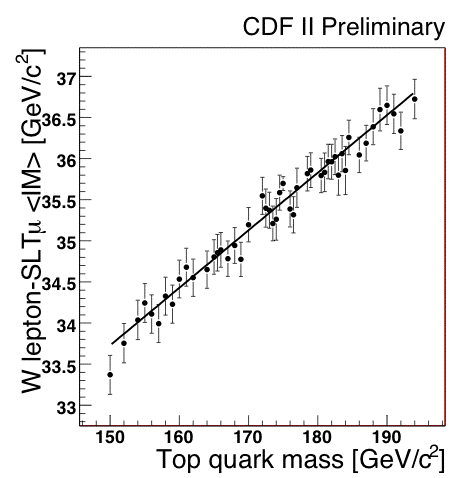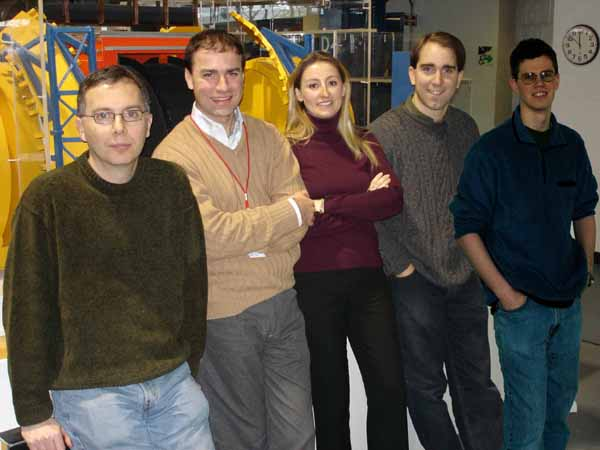## Another pro-LHC top mass measurement October 3, 2008

Posted by dorigo in news, physics, science.
Tags: , , ,

A few months ago I reported here on a CDF technique to measure the mass of the top quark without relying on hadronic jets, whose energy measurement is plagued by many systematic uncertainties. Techniques not relying on the calorimetric measurement of jet energy deposits are quite important for the CMS and ATLAS experiments at the LHC, since the jet energy scale will be very difficult to determine with sufficient accuracy there.

A large statistics of top pair production events is warranted by the 14 TeV proton-proton collisions of the LHC: with respect to the Tevatron, a factor of 100 increase in cross section will be due to the x7 increase in energy, and will be compounded by a factor of 10 increase in instantaneous luminosity.  The rate of production of events of any kind is given by the master formula$\large N = \sigma L$,

where$N$ is the rate, in Hertz, of events produced,$\sigma$ is the cross section responsible for the production, and$L$ is the instantaneous luminosity.

The above means that LHC, after the initial warm-up phase (when energy will be 10 TeV and luminosity will be low), will be producing top quarks at a rate a thousand times higher than what the Tevatron is doing now. This huge statistics will thus allow CMS and ATLAS to extract precise determinations of the top mass from otherwise statistics-limited methods, provided these come with light-weight systematic uncertainties.

And the Tevatron is providing another one proof-of-principle. A new result by CDF uses events selected with a single-lepton topology: one of the top quarks decays to three hadronic jets, the other decays to a jet plus a lepton – neutrino pair. The lepton (an electron or a muon, with a transverse energy larger than 20 GeV) triggers the event collection with high efficiency, and its presence reduces backgrounds quite effectively. After some additional, now standard, selection cuts the data sample contains a large fraction of real top-pair decays. At this point, we note that two of the hadronic jets produced by the fragmentation of quarks emitted in the top decay process are in truth due to b-quarks: each top quark almost always produces a b-quark in its decay, in fact.Just as the top quark may yield a lepton in its decay (see graph on the right, which describes also decays only yielding quarks), through the chain$t \to W b \to l \nu b$ ($l$ stands for the lepton), the b-quark may also decay “semi-leptonically”, as this particular chain is called; in the case of the b-quark, the chain is$b \to W^* c \to l \nu c$, where the superscript asterisk on the W stresses the fact that this particle is virtual, having much less energy than its rest mass.

Because the mass of the b-quark is light with respect to that of the heavy top, all bodies produced in its decay remain within the jet: the b-quark is emitted from the top decay with a large momentum, and the same momentum is imparted to the b daughters, which conserve the original quark direction. The jet will therefore often contain an identified electron or muon. The new technique focuses on these additional, “soft” leptons produced within the hadronic jet, by noting that the invariant mass of the combination between the soft lepton and the primary lepton which triggered the event -the one directly coming from the top quark decay chain- is a kinematical quantity strongly correlated with the mass of the decayed top quark. By measuring the former, one gets information on the latter!Above, the average soft lepton-trigger lepton mass is shown as a function of the top mass, as predicted by a Monte Carlo simulation of top decays with different input mass values. The correlation is linear and well-behaved.Alice Bridgeman, Lucio Cerrito, Ulysses Grundler, and Xiaojian Zhang, a group of physicists from University of Illinois led by Tony Liss (left in the picture; also shown Cerrito, second from left, and Grundler, last on the right), a veteran in CDF with two decades of experience in top quark physics, exploited the above feature with a sample of 2 inverse femtobarns of proton-antiproton collisions. 240 candidate top-pair events, containing about 130 real top-pair decays, were selected to contain a single-lepton topology and a soft-lepton in one of the jets. The invariant mass distribution of the trigger lepton-soft lepton pair resulting from the above selection is shown in the picture below.The black points describe the dilepton mass in the data, the cyan histogram is the background contribution, and the blue line shows the likelihood fit. The inset shows the likelihood values as a function of the unknown top mass value. The minimum is found at$M_t = 181.3 \pm 12.4 \pm 3.5 GeV/c^2$: the first uncertainty is statistical, and it shows that the method is not useful at the Tevatron -where CDF and D0 have already measured the top mass with an uncertainty of less than two GeV. However, the systematic uncertainty is much smaller, and it can still be reduced by more accurate studies. Most importantly, systematic effects due to the measurement of the jets in the calorimeter are totally avoided by this technique.

More information on this particular analysis is available in the public page of the measurement.